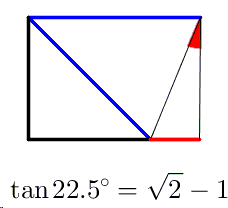# Tangent of 22.5° - Proof Wthout Words

Here's a proof without words by Grégoire Nicollier from Sion, Switzerland.Grégoire notes that the proof can be directly folded from the standard A4 paper sheet (whose dimensions $11.7''\times 8.3''$ or in metric millimeters $297×210$) have been specifically designed to approximate $\sqrt{2}\times 1$.Paper Folding Geometry### Trigonometry